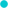# Calculate Calories Needed for Weight Loss#Weight Loss Calculator

An online weight loss calculationConvert Pounds to KG / KG to Pounds## How Weight loss calculator works ?

This will Calculate Calories Needed for Weight Loss.One pound or 0.45 Kilograms of body fat equals about 3,500 calories.To lose a pound, you need to burn 3500 more calories than you take in.

Example, Over seven days, if you burn of 500 to 1,000 calories a day yields a weight loss of about 1 to 2 pounds a week.

##Age Calculators ►

Horse Age Calculator , Cow Age Calculator , Cat Age Calculator , Dog Age Calculator, Human Age Calculator 100 Days Old Calculator Date Difference Calculator

##Health Calculators